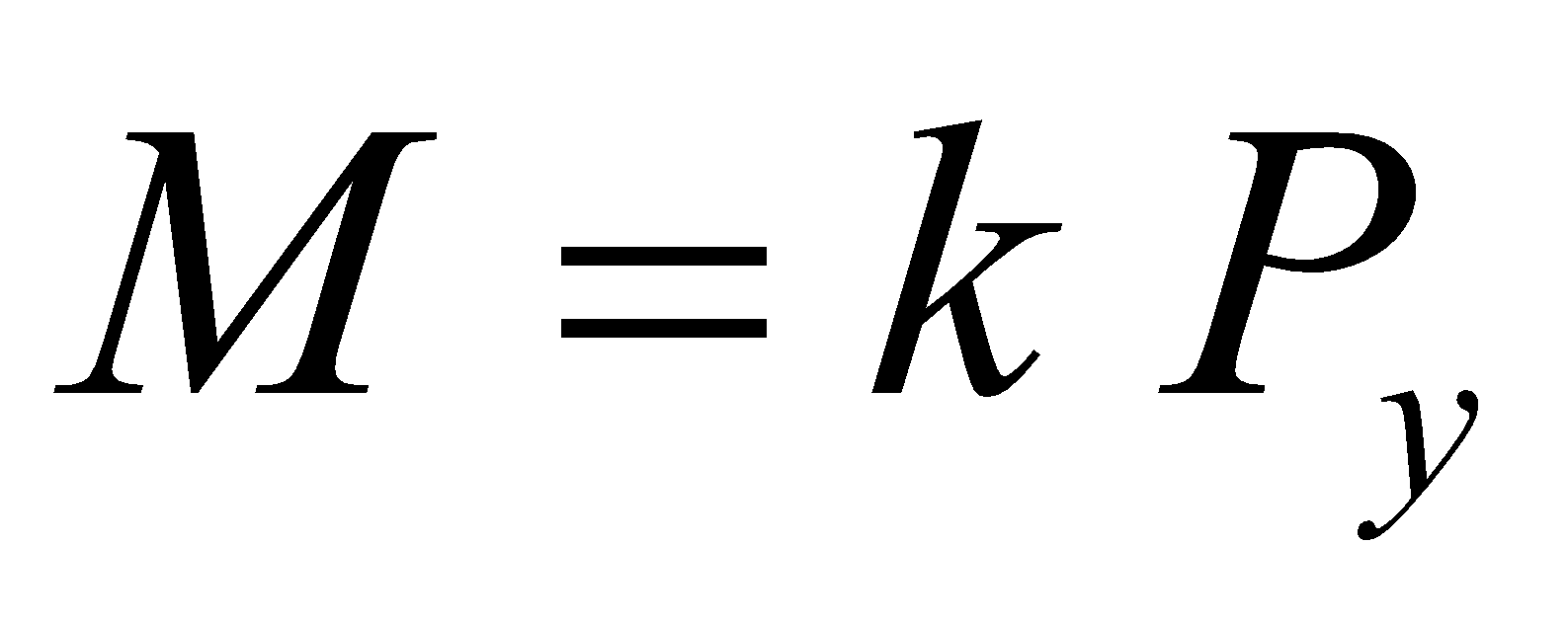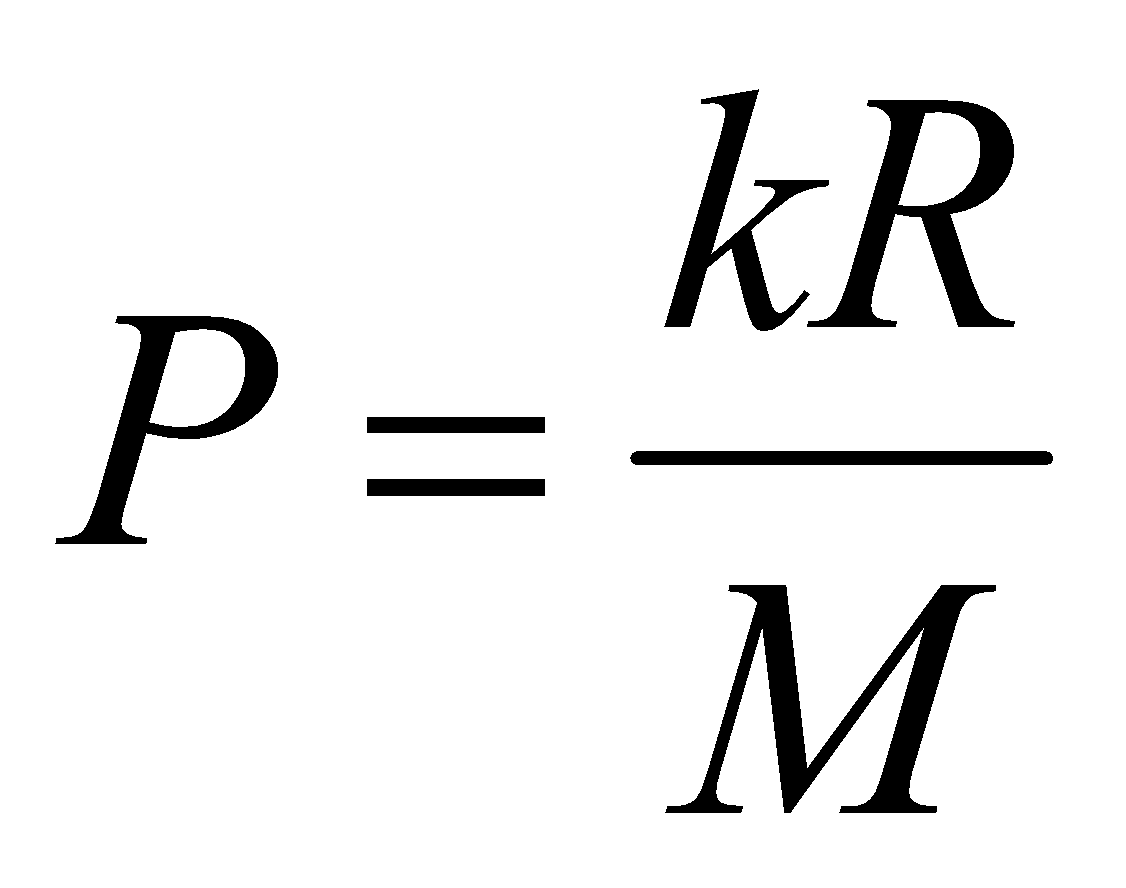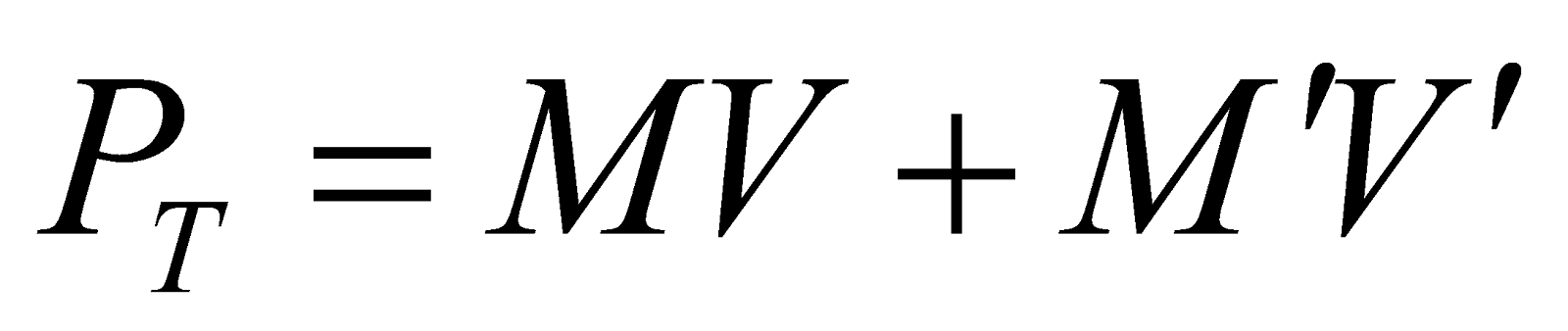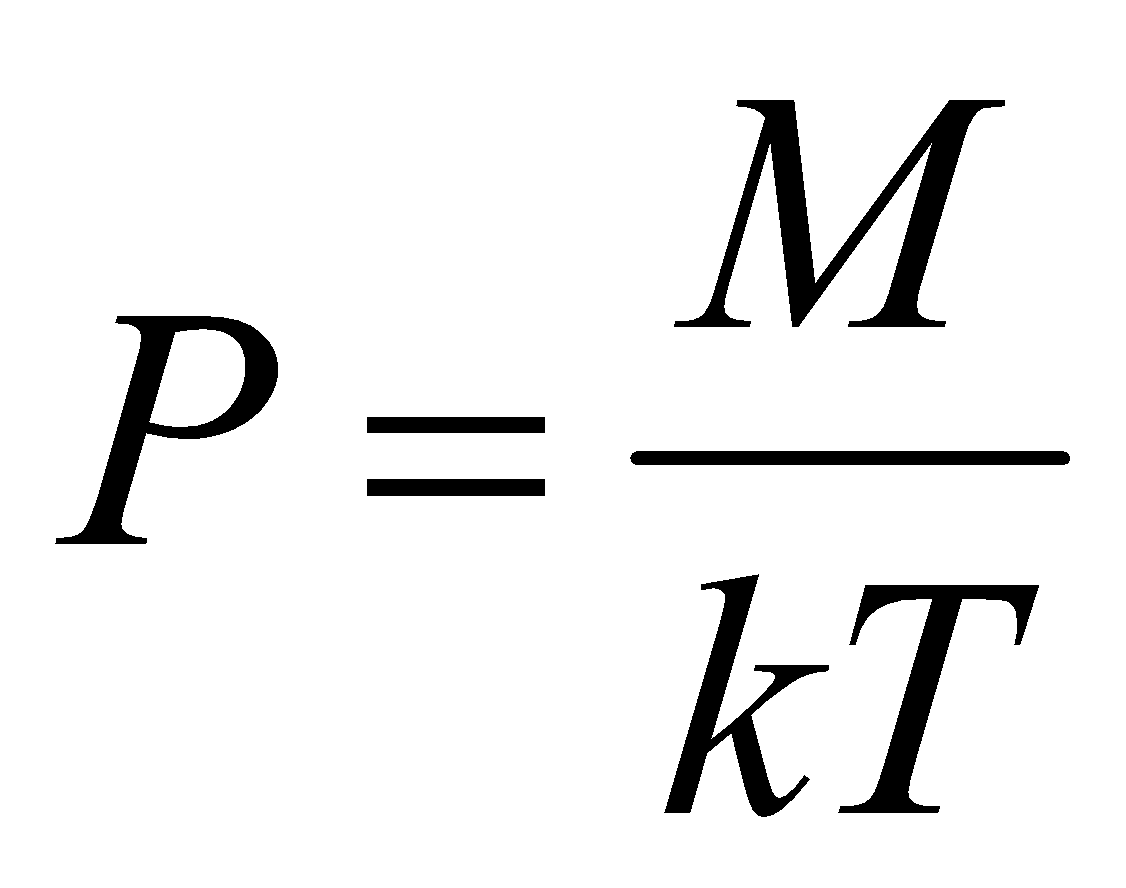## Thursday, January 03, 20192013
(May)
ECONOMICS
(General)
Course: 201
(Macroeconomics)
Full Marks: 80
Pass Marks: 32
Time: 3 hours
The figures in the margin indicate full marks for the questions

1. Define gross national product.
2. “Supply creates its own demand”.
The statement was propounded by
1. J. S. Mill.
2. David Ricardo.
4. J. B. Say.
1. The Quantity Theory of Money given by Fisher is expressed by the equation.
1.2.3.4.1. According to Keynes, “Effective demand is determined by aggregate supply function and aggregate demand function”. (Write True or False)
2. Which is not a function of commercial bank?
1. Credit creation.
2. Accepting deposit.
4. Issue of paper currency.
1. During inflation, the condition of fixed income earners are
2. Benefited all.
3. Remaining same.
4. None of the above.
1. Which of the following is the banker of the government and banks?
1. Commercial bank.
2. Cooperative bank.
3. Central bank.
4. Industrial bank.
1. Modern theory of international trade was propounded by
1. Ricardo.
2. Haberler.
3. Heckscher-Ohlin.
2. Write short notes on any four (within 150 words each): 4x4=16
1. Marginal Propensity to Consume (MPC).
2. Gross national product.
3. Difference between commercial bank and central bank.
4. Marginal Efficiency of Capital (MEC)
6. Inflation.
Answer the following questions (within 500 words each):
3. (a) Discuss different methods of computing national income. What are the problems for computing national income in India? Name the organization which is associated with the computation of national income in India. 6+5+1=12
Or
(b) What is macroeconomics? Explain the main characteristics of macroeconomics, by stating its limitations. 2+10=12
4. (a) Explain various functions of money. What is near money? 10+1=11
Or
(b) Critically discuss the Cambridge version of the quantity theory of money. 11
5. (a) What is commercial bank? What are the functions of commercial bank? Discuss. Name a major public sector commercial bank in India. 1+9+1=11
Or
(b) Discuss various methods of credit control by the central bank of a country. 11
6. (a) Explain the classical theory of employment. Mention its limitations. 8+3=11
Or
(b) What is consumption function? Analyze the determinants of consumption function. 2+9=11
7. (a) What is international trade? Explain the fundamental differences between international trade and inter-regional trade. 2+9=11
Or
(b) Analyze the Purchasing Power Parity theory. 11

***